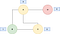FIFO vs LIFO

# Audience

This article is aimed at engineers with a small amount of programming experience, who are looking for their first introduction to stacks and queues. Alternatively it can be used by more experienced engineers as a light refresher on the subject.

It will help if you have seen graphs before, as we will be using them to demonstrate some of the applications of stacks and queues. We also use linked lists for our queue and stack implementations!

If you need to brush up on either of these subjects, you can use my article for graphs here, and my article for linked lists here.

# Argument

Stacks and queues are both linear data structures with flexible sizes (they aren’t initialised to a set capacity like an array). The difference between the two is the way we add and retrieve elements.

• Stacks are Last In First Out (LIFO): The last element we put on the stack is the one we take off first.
• Queues are First in First Out (FIFO): We take elements from the queue in the same order we put them on.

We can think about this using real life stacks and queues. If I have a stack of papers, the last paper I put on the pile is the first one I will take from the top. Similarly, if I’m queuing to get into a film, the person who arrived first will be let in first.

Let’s look at how we might implement both of these ideas. Our queue will have four methods:

1. `isEmpty`, used to check if the queue has items or not.
2. `peek`, used to look at the next item in the queue (but not remove it).
3. `add`, used to add a new item onto the queue.
4. `remove`, used to take an item from the queue.

The code is implemented below.

We can do a similar thing for stacks. Our stack will also have four methods.

1. `isEmpty`, used to check if the queue has items or not.
2. `peek`, used to look at the next item in the stack (but not remove it).
3. `push`, used to add a new item onto the stack.
4. `pop`, used to take an item from the stack.

Now we have covered the definition of stacks and queues, and how we may implement them, let’s look at one of their most common uses: searching. These will be done in the context of searching in a graph. There are two ways this can be done.

1. Breadth First Search (BFS): In this technique we explore the graph level by level.
2. Depth First Search (DFS): In this technique we go as far down each path as we can before going back up.

Let’s start with BFS, which uses a queue. We can demonstrate this with the below diagram.We start at node B, then visit nodes A and C, then node D

The above diagram represents a BFS starting at node `B`. We then visit nodes `A` and `C`, then finally node `D`. You see we go down the graph in order of the node’s depth. We represent this in the coded solution below.

From the above we can see that the queue’s FIFO property means we will process nodes in the order they are added, i.e. layer by layer.

Depth first search is different in that we go all of the way to the end of a path before recursing back up and exploring the next one.

We start at `B`, then go through to `A`, then to `D`. This marks the end of our current possible routes, so we return to `B` and look for the next one. The only remaining node is `C`, so we follow that path all the way down. As we have seen `A` before, we do not visit it, and that’s us done!

DFS is interesting as it can be implemented in two ways: using recursion and the implicit call stack, or using an explicit stack. Let’s examine both.

The below is the commented, recursive implementation of DFS.

We can then extend this to use an explicit stack.

# Conclusion

In conclusion we have introduced the concept of stacks and queues, their implementation, and concluded with their example usage.

Principal Software Engineer at the BBC